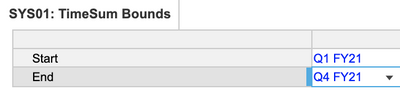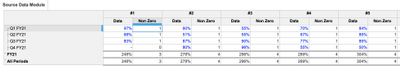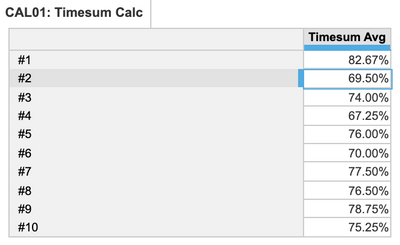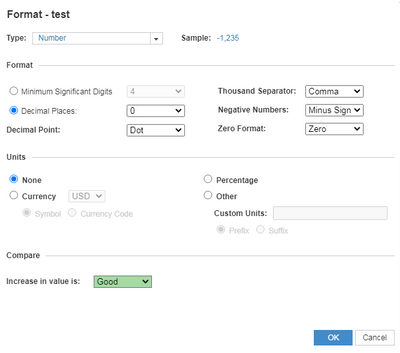# Exclude Zeros in Time Sum AverageHi,

I am using a TIMESUM average and I'm wondering if there is a way to exclude zeros from the average. Formula: TIMESUM(Build Factor, TIME.'Q2 FY16', TIME.'Q4 FY19', AVERAGE) Pictures attached.

Tagged:

•Hi @nawalker56 ,

First of all, try not to use hard coded time references. There are multiple potential issues if you write the formula like that. Instead, use a module to define the start and end of the timesum and then use that (see image below).For the average calculation, in the source module use another line item to mark a data point to be 1 or 0 depending on whether it is non zero or not. Then, in your destination calc module, use two timesums - one to sum the data and one to sum the non zero data point count and divide the first one by the second. The below screenshots and formula should help:

Non zero Data:

`IF Data <> 0 THEN 1 ELSE 0`

Final Formula:

`TIMESUM(Source Data Module.Data, 'SYS01: TimeSum Bounds'.Start, 'SYS01: TimeSum Bounds'.End) / TIMESUM(Source Data Module.Non Zero, 'SYS01: TimeSum Bounds'.Start, 'SYS01: TimeSum Bounds'.End)`Hope this helps!

•set format to 0 decimal placesand if still you want to hide the zero values then apply filter >0.